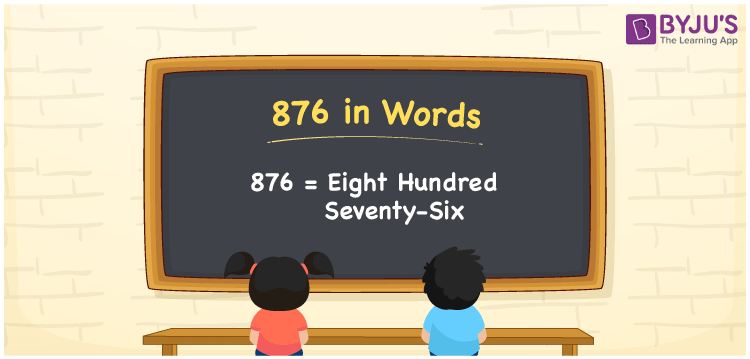# 876 in Words

876 in words is expressed as “Eight hundred seventy-six”. In Mathematics, 876 is a cardinal number and it has three-digits. For example, if you spent Rs. 876 on purchasing chocolates, you can write it as “I spent Rs. Eight hundred seventy-six on purchasing chocolates”. Now, let us discuss how to write the number 876 in words using the place value system in detail.

 876 in Words: Eight Hundred Seventy-six. Eight Hundred Seventy-six in Numerical Form: 876.

## 876 in English Words## How to Write 876 in Words?

The place values of 876 is presented in the below table.

 Hundreds Tens Ones 8 7 6

The expanded form of 876 is as follows:

= 8 × Hundred + 7 × Ten + 6 × One

= 8 × 100 + 7 × 10 + 6 × 1

= 800 + 70 + 6

= 876

= Eight hundred seventy-six

Hence, 876 in words is eight hundred seventy-six.

The number 876 is a natural number and it lies between 875 and 877.

876 in words – Eight hundred seventy-six

Is 876 an odd number? – No

Is 876 an even number? – Yes

Is 876 a perfect square number? – No

Is 876 a perfect cube number? – No

Is 876 a prime number? – No

Is 876 a composite number? – Yes

## Frequently Asked Questions on 876 in Words

Q1

### Write 876 in words.

876 in words is eight hundred seventy-six.

Q2

### Simplify 800 + 76, and express it in words.

Simplifying 800 + 76, we get 876. Hence, 876 in words is eight hundred seventy-six.

Q3

### Is 876 a composite number?

Yes, 876 is a composite number.